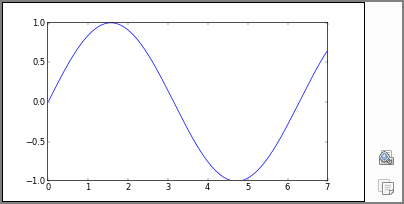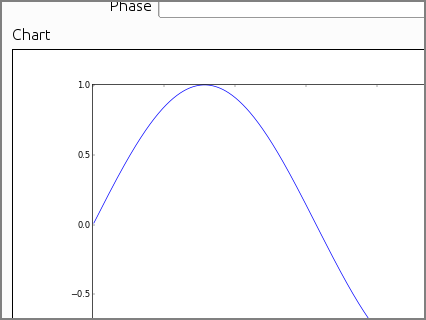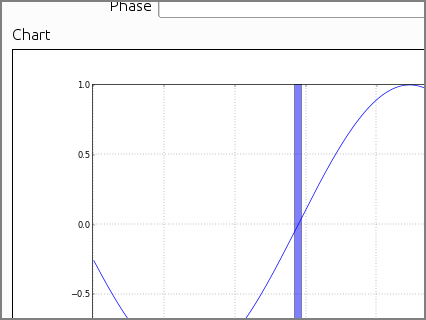# Charts¶

To enable charts, Camelot is closely integrate with Matplotlib, one of the very high quality Python charting packages.

Often creating a chart involves gathering a lot of data, this needs to happen inside the model, to free the GUI from such tasks. Once the data is gathered, it is put into a container, this container is then shipped to the gui thread, where the chart is put on the screen.## A simple plot¶

As shown in the example below, creating a simple plot involves two things :

1. Create a property that returns one of the chart containers, in this case the PlotContainer is used.
2. Specify the delegate to be used to visualize the property, this should be the ChartDelegate
```from camelot.admin.object_admin import ObjectAdmin
from camelot.view.controls import delegates
from camelot.container.chartcontainer import PlotContainer

class Wave(object):

def __init__(self):
self.amplitude = 1
self.phase = 0

@property
def chart(self):
import math
x_data = [x/100.0 for x in range(1, 700, 1)]
y_data = [self.amplitude * math.sin(x - self.phase) for x in x_data]
return PlotContainer( x_data, y_data )

form_display = ['amplitude', 'phase', 'chart']
field_attributes = dict(amplitude = dict(delegate=delegates.FloatDelegate,
editable=True),
phase = dict(delegate=delegates.FloatDelegate,
editable=True),
chart = dict(delegate=delegates.ChartDelegate) )
```

The PlotContainer object takes as its arguments, the same arguments that can be passed to the matplotlib plot command. The container stores all those arguments, and later passes them to the plot command executed within the gui thread.The simpel chart containers map to their respective matplotlib command. They include :

class camelot.container.chartcontainer.PlotContainer(*args, **kwargs)[source]
class camelot.container.chartcontainer.BarContainer(*args, **kwargs)[source]

## Actions¶

The PlotContainer and BarContainer can be used to print or display charts as part of an action through the use of the appropriate action steps :

```        class ChartPrint( Action ):

def model_run( self, model_context ):
from camelot.container.chartcontainer import BarContainer
from camelot.view.action_steps import PrintChart
chart = BarContainer( [1, 2, 3, 4],
[5, 1, 7, 2] )
print_chart_step = PrintChart( chart )
print_chart_step.page_orientation = QtGui.QPrinter.Landscape
yield print_chart_step
```

For more advanced plots, the camelot.container.chartcontainer.AxesContainer class can be used. The AxesContainer class can be used as if it were a matplotlib Axes object. But when a method on the AxesContainer is called it will record the method call instead of creating a plot. These method calls will then be replayed by the gui to create the actual plot.

```from camelot.admin.object_admin import ObjectAdmin
from camelot.view.controls import delegates
from camelot.container.chartcontainer import AxesContainer

class Wave(object):

def __init__(self):
self.amplitude = 1
self.phase = 2.89

@property
def chart(self):
import math
axes = AxesContainer()
x_data = [x/100.0 for x in range(1, 700, 1)]
y_data = [self.amplitude * math.sin(x - self.phase) for x in x_data]
axes.plot( x_data, y_data )
axes.grid( True )
axes.axvspan(self.phase-0.05, self.phase+0.05, facecolor='b', alpha=0.5)
return axes

form_display = ['amplitude', 'phase', 'chart']
field_attributes = dict(amplitude = dict(delegate=delegates.FloatDelegate,
editable=True),
phase = dict(delegate=delegates.FloatDelegate,
editable=True),
chart = dict(delegate=delegates.ChartDelegate) )
```## More¶

For more information on the various types of plots that can be created, have a look at the Matplotlib Gallery.

When the AxesContainer does not provide enough flexibility, for example when the plot needs to manipulated through its object structure, more customization is possible by subclassing either the camelot.container.chartcontainer.AxesContainer or the camelot.container.chartcontainer.FigureContainer :